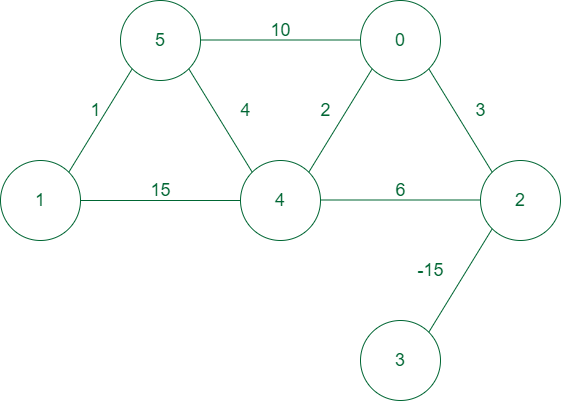GeeksforGeeks App
Open AppBrowser
Continue

## What is Weighted Graph?

A weighted graph is defined as a special type of graph in which the edges are assigned some weights which represent cost, distance, and many other relative measuring units.Example of a weighted graph

### Applications of Weighted Graph:

• 2D matrix games: In 2d matrix, games can be used to find the optimal path for maximum sum along starting to ending points and many variations of it can be found online.
• Spanning trees: Weighted graphs are used to find the minimum spanning tree from graph which depicts the minimal cost to traverse all nodes in the graph.
• Constraints graphs: Graphs are often used to represent constraints among items. Used in scheduling, product design, asset allocation, circuit design, and artificial intelligence.
• Dependency graphs: Directed weighted graphs can be used to represent dependencies or precedence order among items. Priority will be assigned to provide a flow in which we will solve the problem or traverse the graph from highest priority to lowest priority. Such graphs are often used in large projects in laying out what components rely on other components and are used to minimize the total time or cost to completion while abiding by the dependencies.
• Compilers: Weighted graphs are used extensively in compilers. They can be used for type inference, for so-called data flow analysis, and many other purposes such as query optimization in database languages.
• Artificial Intelligence: Weighted graphs are used in artificial intelligence for decision-making processes, such as in game trees for determining the best move in a game.
• Image Processing: Weighted graphs are used in image processing for segmentation, where the weight of the edges represents the similarity between two pixels.
• Natural Language Processing: Weighted graphs are used in natural language processing for text classification, where the weight of the edges represents the similarity between two words.

### Real-Time Applications of Weighted Graph:

• Transportation networks: Using weighted graphs, we can figure things out like the path that takes the least time, or the path with the least overall distance. This is a simplification of how weighted graphs can be used for more complex things like a GPS system. Graphs are used to study traffic patterns, traffic light timings and much more by many big tech companies such as OLA, UBER, RAPIDO, etc. Graph networks are used by many map programs such as Google Maps, Bing Maps, etc.
• Document link graphs:  Link weighted graphs are used to analyze relevance of web pages, the best sources of information, and good link sites by taking the count of the number of views as weights in the graph.
• Epidemiology: Weighted graphs can be used to find the maximum distance transmission from an infectious to a healthy person.
• Graphs in quantum field theory: Vertices represent states of a quantum system and the edges represent transitions between them. The graphs can be used to analyze path integrals and summing these up generates a quantum amplitude. Research to find the maximum frequency along a path can be done using weighted graphs.
• Social network graphs: We can find which all users are connected in a network both directly(direct connection) and indirectly(indirect connection). But now weighted graphs are also used in social media for many purposes, for example, In recent times Instagram is using features like close friends which is not the same as all friends these features are being implemented using weighted graphs.
• Network packet traffic graphs: Network packet traffic graphs are used for analyzing network security, studying the spread of worms, and tracking criminal or non-criminal activity.

• Better representation of real-world scenarios: Weighted graphs are a more accurate representation of many real-world scenarios, where the relationships between entities have varying degrees of importance. For example, in a road network, some roads may have higher speed limits or more lanes, and these differences can be represented using weights.
• More accurate pathfinding: In a weighted graph, finding the shortest path between two nodes takes into account the weights of the edges, which can lead to more accurate results. This is particularly useful in applications where finding the optimal path is critical, such as in logistics or transportation planning.
• More efficient algorithms: Many graph algorithms are more efficient when applied to weighted graphs, such as Dijkstra’s algorithm for finding the shortest path. This is because the weights provide additional information that can be used to optimize the search.
• More flexible analysis: Weighted graphs allow for more flexible analysis of the relationships between nodes. For example, it is possible to calculate the average weight of edges, or to identify nodes with unusually high or low weights. This can provide insights into the structure of the graph and the relationships between its nodes.
• Ability to model uncertainty: Weighted graphs can be used to model uncertain or probabilistic relationships between nodes. For example, in a social network, the weight of an edge could represent the probability of a connection between two people, rather than a binary “friend” or “not friend” relationship.